# M8-S5: The Electron

• ### investigate, assess and model the experimental evidence supporting the existence and properties of the electron, including:

– early experiments examining the nature of cathode rays

– Thomson’s charge-to-mass experiment

– Millikan's oil drop experiment (ACSPH026)

### What are cathode rays?

• Cathode rays were produced in cathode ray tubes which are vacuum tube is a discharge tube consisting of two electrodes enclosed within a sealed glass or metallic chamber, from which the air has been withdrawn to create a near-vacuum environment.
• Investigation of vacuum tubes could not occur until good vacuum pumps had been invented.
• By the end of the 19th Century, vacuum pumps were perfected, and glass tubes were being made.
• The positive electrode is the anode, and the negative electrode is the cathode. When a high voltage is connected between the electrodes, an invisible ray (cathode ray) travels from the cathode to the anode.
• The high voltage is essential to overcome the poor conductivity of a near vacuum environment (most likely gas).• Under normal conditions of temperature and pressure, air acts as an insulator. However, at reduced pressure, air becomes conductive.

Are cathode rays charged particles or electromagnetic waves?

• After Cathode Ray was discovered in 1855, many experiments were done with them in order to understand their nature.
• Observations from the experiments confused some scientists as the rays showed properties of both the wave and particles.
• These observations were as follows:

 Charged Particle Properties Electromagnetic Wave Properties Can be deflected by magnetic fields They are identical, regardless of material used Can be deflected by electric field It emanates from the cathode and travels in a straight line The rays carry energy and momentum Would cast a shadow of a solid object Are attracted to positive charges Could penetrate thin metal foils

Cathode ray tubes allow the manipulation of a stream of charged particles

• High voltages applied to the electrodes of a cathode ray tube results in cathode rays flowing from the cathode (-) to the anode (+).
• At different air pressures, different bright effects appear in the tubes for example bands, striations and dark spaces. These observations are results of spectroscopy.
• These are caused by cathode rays striking atoms in the air inside the tube.
• The atoms become excited then release photons of visible light.
• Cathode ray tubes allowed the manipulation of a stream of charged particles:
• Electrical and magnetic fields can be placed inside or outside the tube to deflect the rays.
• Solid objects such as a paddle wheel placed inside the tube can block the ray off.### Experiments involving cathode ray tubes

Maltese cross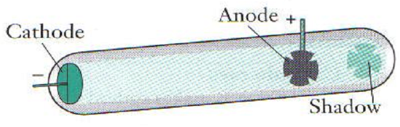• A maltese cross was placed in the path of the cathode ray in a cathode ray tube.
• A perfect shadow of the maltese cross was formed on the far end of the tube, indicating that cathode rays travel in straight lines like light.
• Similar to light, cathode rays can also cast shadows

Electric plates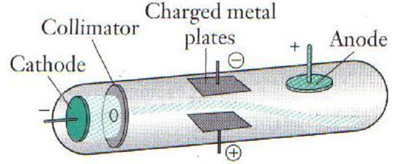• With electric plates, the rays always were deflected towards the positive plate, indicating that the cathode ray has a negative charge.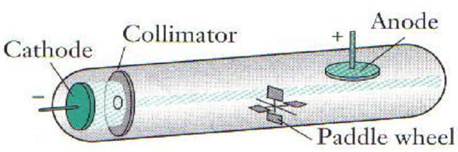• When the glass wheel was struck by the cathode ray, the glass wheel slowly rotated, indicating that the cathode rays have momentum and therefore mass. This supported the particle side of the controversy as electromagnetic waves are massless.
• It also showed that the ray was produced from the cathode.

### J.J. Thomson’s Experiment (1897)

• Experimental aim: Thomson, through this experiment, calculated the charge to mass ratio (q/m) of cathode rays.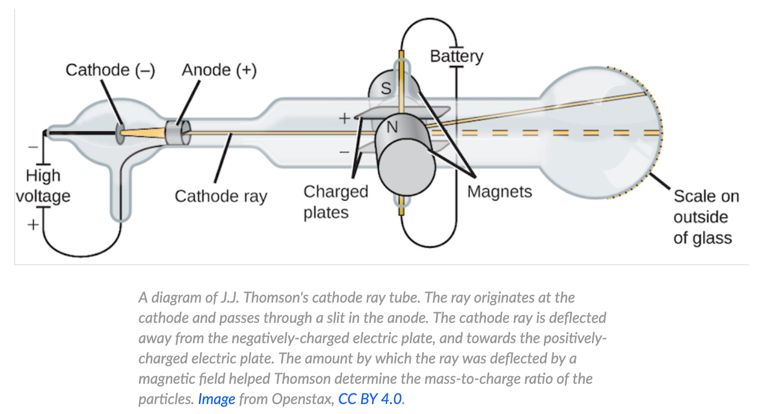• Thomson’s experiment involved manipulating cathode ray path through the use of electric and magnetic fields.
• A charge particle can be deflected by electric fields and magnetic fields.
• The particle will be deflected by a force in the electric field.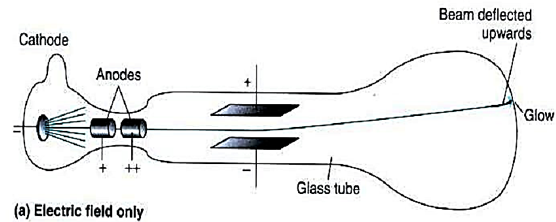• The particle will be deflected by a force in the magnetic field• If the magnitude of forces in the magnetic field and the electric field are equal and their directions opposite, then the particle will not be deflected.• Thomson performed an experiment using cathode rays to determine the ratio of the charge of cathode rays to their mass.
• Thomson used a modified version of a cathode ray tube. He placed electric and magnetic fields at right angles to each other so that their forces would completely negate each other. A fluorescent screen showed where the particle hit.
• When the particle is deflected by the electric field, it experiences a force F=qE
• When deflected by the magnetic field, it moves in the arc of a circle. The force is given by F=qvB
• By equating the centripetal force and the force experienced by the magnetic field, he found:• Thomson then removed the electric field and applied only the magnetic field, so that the cathode ray would deflect and the radius of the path could be physically determined.
• Equating the magnetic force to the electric force:• By substituting the value for v: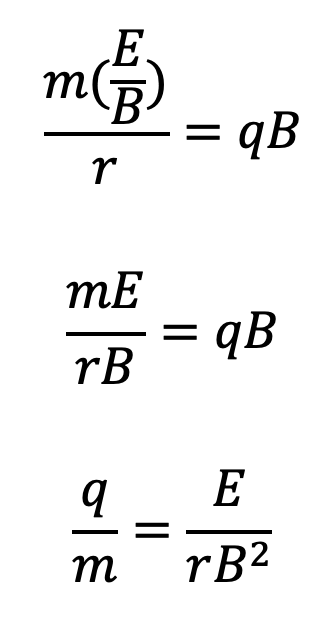• Results
• The charge/mass ratio was determined to be 1.76 x 1011 Ckg–1.
• This ratio was the same for all materials used.
• The q/m ratio of cathode rays to be 1800 times greater than for hydrogen ions (protons), meaning the mass of cathode ray particles is 1800 times smaller.
• This q/m ratio was crucial in showing that the atom is divisible and that cathode rays were subatomic particles - electrons.

### Millikan’s Oil-drop experiment (1909)

• Robert Millikan utilised Newtonian physics and small oil droplets to determine the charge of an electron – a feat that Thomson could not previously achieve.• Millikan used an atomiser to spray droplets of oil into a chamber in which the oil droplets eventually would fall into a strong electric field.
• Within this field (between charged metal plates), X-ray was used to ionise gas molecules which produced free electrons that attached themselves to the oil droplets. Thus, the oil droplets acquired negative charge
• The electric field was orientated such that the positive terminal was at the top. Therefore, the electric force that acts on the oil droplets would have an upward direction.
• At the same time, oil droplets would also experience downward gravitational force, upward buoyance force and drag force (direction depends on velocity of oil droplets).
• By manipulating the voltage (strength of the electric field), Millikan was able to determine the electric charge of the electron.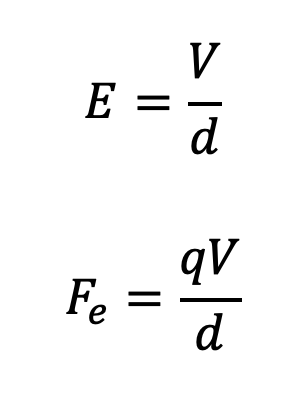By equating the magnitude of electric and gravitational forces, the droplets will hover.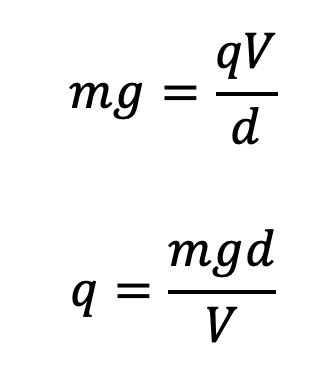Where m is the mass of an oil droplet, g is the gravitational acceleration, d is the distance between the parallel metal plates which produced the electric field and V is the voltage applied to the plates.• Millikan used an ingenious method to calculate the mass of oil droplets. He measured the terminal velocity of oil droplets when the electric field is switched off. When the electric force is absent, the oil droplets are acted on by gravitational and drag force (friction caused by air). Since drag force is influenced by the droplets’ diameter, Millikan was able to use their velocity to calculate the radius and hence their volume.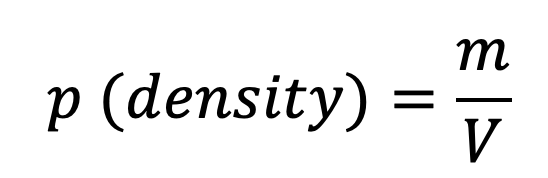• Using the calculated volume (which is different among droplets) and the density of oil, he was able to calculate the mass of the oil droplets. You will not be required to calculate mass this way, all calculation-based questions will provide the droplets’ mass or its density and radius/diameter.
• Subsequently, Millikan calculated the charge for numerous droplets, each different in mass (enhanced reliability of results)
• Results – Millikan that the charges of differently sized droplets were quantised in the sense that they are multiples of a certain number. This number was determined to be 1.59 x 10-19 which was deemed to be the electric charge of an electron.
• Millikan’s electric charge determination was very accurate given that the current accepted value is 1.602 x 10-19 It was later discovered that the experimental value deviated from the actual value because the viscosity of air was inaccurately calculated at the time (viscosity of air affected the buoyancy force).

Summary

• Thomson determined the cathode ray (electron)’s charge to mass ratio which proved that it was a particle rather than a wave.
• Millikan determined the charge of the electron with very high accuracy and showed that charge is quantised.

Practice Question 1

(a) Explain the conditions that are required to emit cathode rays. (1 mark)

(b) Describe a simple experiment using discharge tubes that shows the particle nature of cathode rays. (2 marks)

Practice Question 2

Explain how Thomson was able to settle the debate between the particle and wave properties of cathode rays. (2 marks)

Practice Question 3

In one version of the Millikan oil-drop experiment, the mass of an oil drop is 6.8 x 10-15 kg and a potential difference of 7.2 x 104 V is applied across plates that are separated by 52 cm. The oil drops fall with uniform velocity.

(a) Determine the charge of the oil drop. (2 marks)
(b) How many electrons are there in the oil droplet? (1 mark)

Previous Section: Energy Sources of Stars

Next Section: Rutherford's Atomic Model